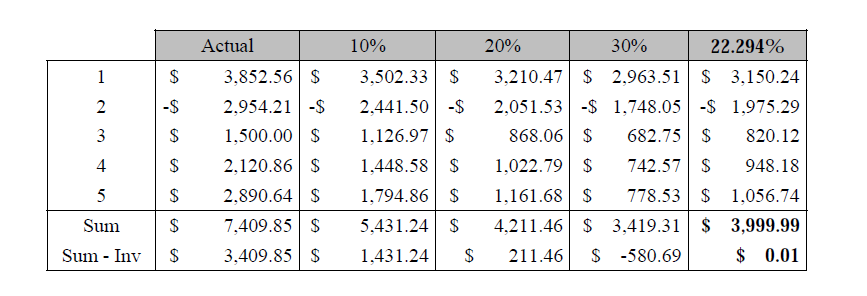# 2840. Calculating IRR

Johnny Money is a big-time investor well-known for having the ability to identify good business opportunities. To measure the profitability of a business or project, Johnny calculates the internal rate of return (IRR) based on a projected or existing cash-flow of such a business. If the IRR is greater than any interest rate offered by the bank, the investment is convenient.

In more specific terms, the IRR of an investment is the interest rate (%) at which the sum of the present value of all projected costs (negative cash flows) and benefits (positive cash flows) of the investment equals the initial investment. The present value is calculated dividing the value by an interest rate as many times as the period indicates. For example, the present value of a cost of $100.00 projected for the 3rd period (or month) with an interest rate of 10% is$75.13.

The problem consists of calculating the IRR given an initial investment and the cash flow. For a better understanding of the problem, consider the following example. The initial investment is $4,000.00 and five periods are projected. The first one results in a benefit of$3,852.56; the second period projects a cost of $2,954.21; the three following periods projects benefits of$1,500.00, $2,120.86 and$2,890.64, respectively. Was it a profitable investment? To answer that, we need to find an interest rate such that the sum of the present values equals the initial investment. From the table above, it is clear that such an interest rate is 22.294%, from which we conclude that Johnny Money will accept to invest in this business.The reader is suggested to treat this problem as a root-finding problem, which can be solved by means of numerical methods, particularly, the bisection method. This method repeatedly cuts the current interval in half, defining two new subintervals; then it selects the one in which the root must lie: the functions of the first and last values of the subinterval have different signs. Assume that every IRR ranges from -100% to 100%. As an initial interval, the positive half if the sum of the projected values (in the table, \$7,409.95) is greater than the investment; choose the negative half, otherwise.

### 输入格式

The first line contains an integer number, N > 0, denoting the number of test cases. The next N lines contain each a cash flow defined as a space-separated list of two-decimal numbers denoting costs (if negative) or benefits (if positive).

### 输出格式

The output consists of N lines, where each line k <= N contains a three-decimal number in the range (-100.0.. 100.0) followed by the character %, denoting the estimated IRR for cash flow k.

### 样例

Input
3
500.00 75.60 174.40 -60.75 319.25 84.63 31.17
100.00 42.27 35.00 -22.35 10.00
4000.00 3852.56 -2954.21 1500.00 2120.86 2890.64

Output
6.873%
-26.369%
22.294%
Hint: If there are more than 3 decimals in the output, it has to be rounded using the round-up algorithm.


10 人解决，21 人已尝试。

10 份提交通过，共有 117 份提交。

7.3 EMB 奖励。